Ex 6.3

Chapter 6 Class 10 Triangles (Term 1)
Serial order wise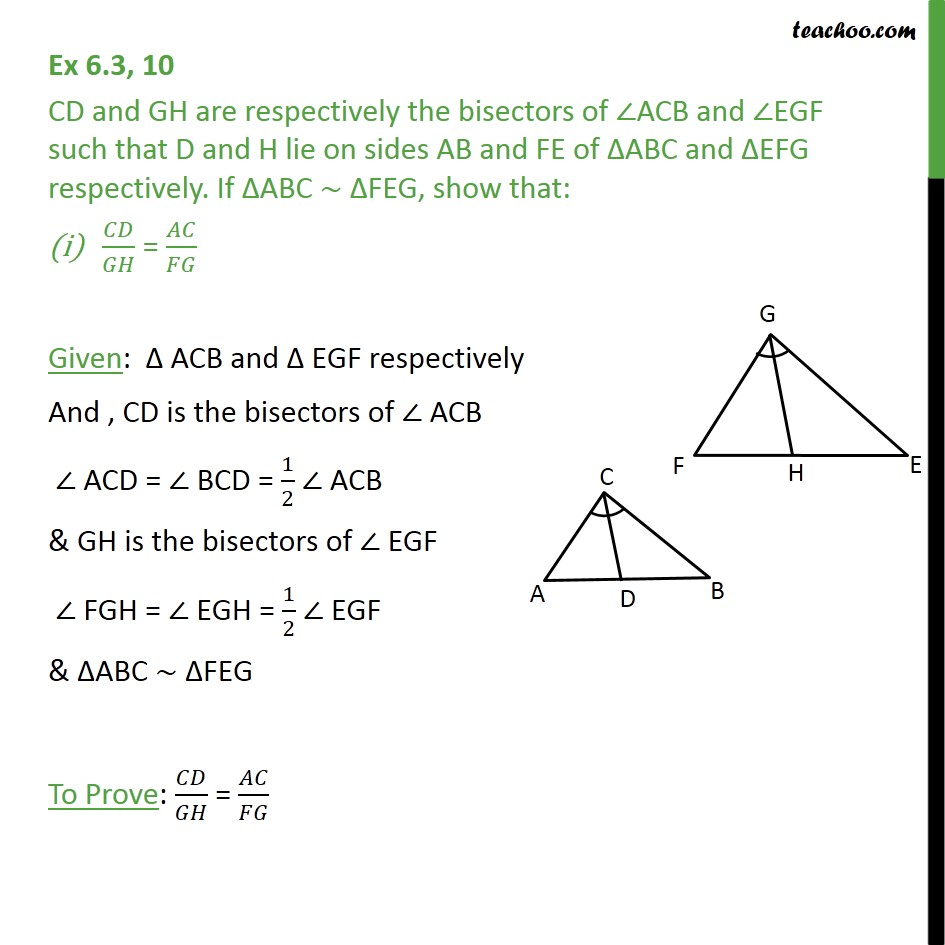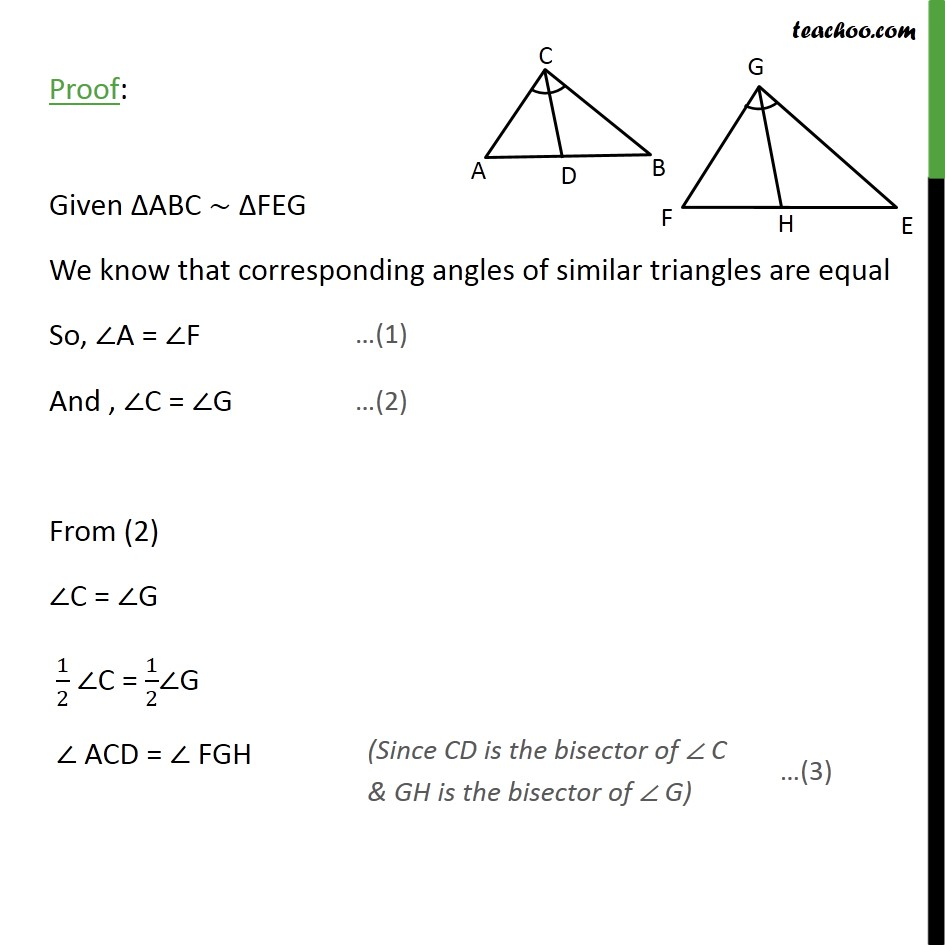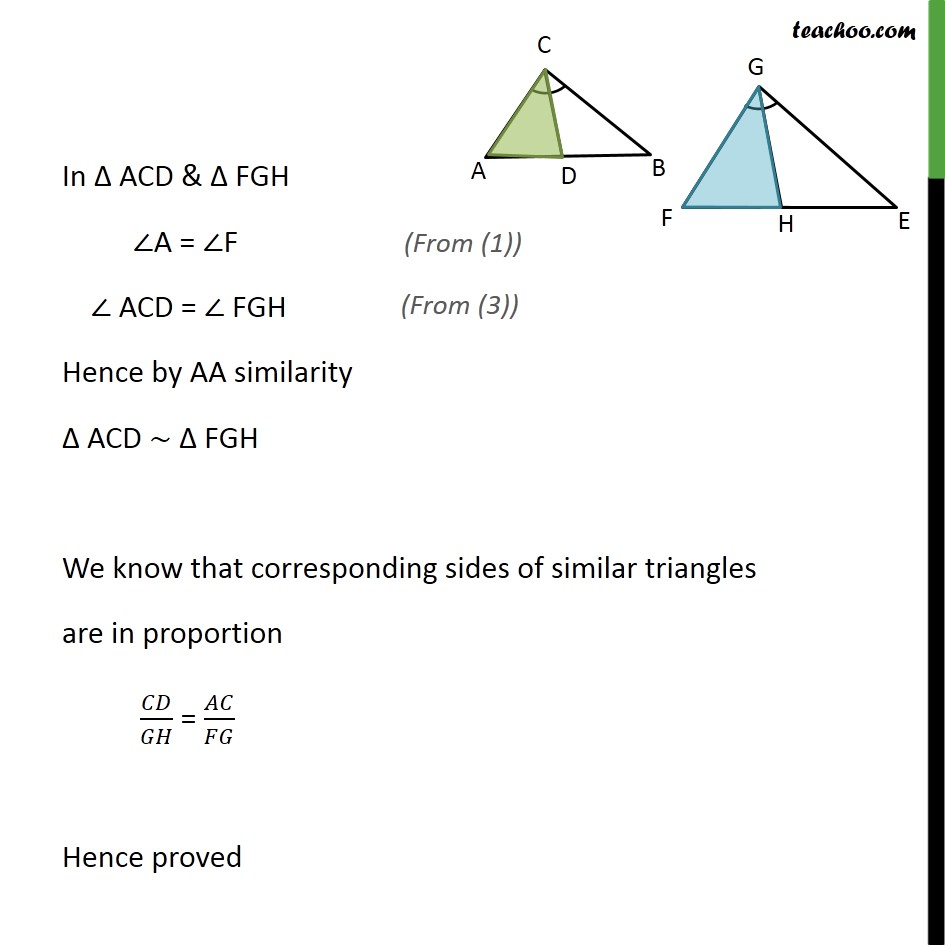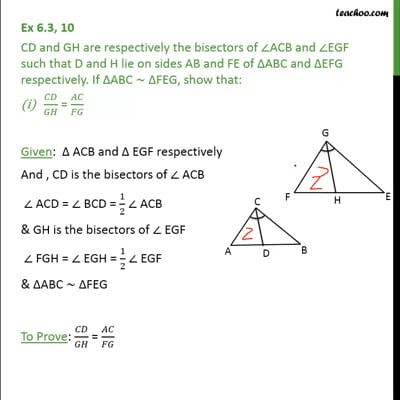This video is only available for Teachoo black users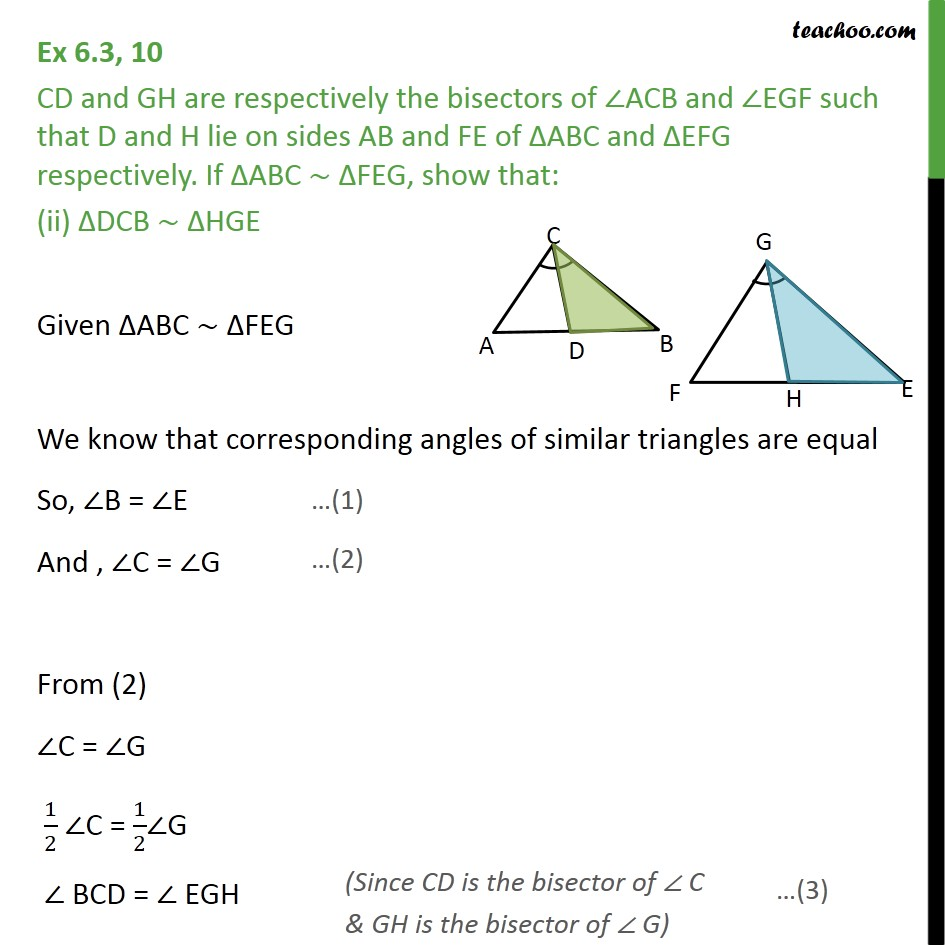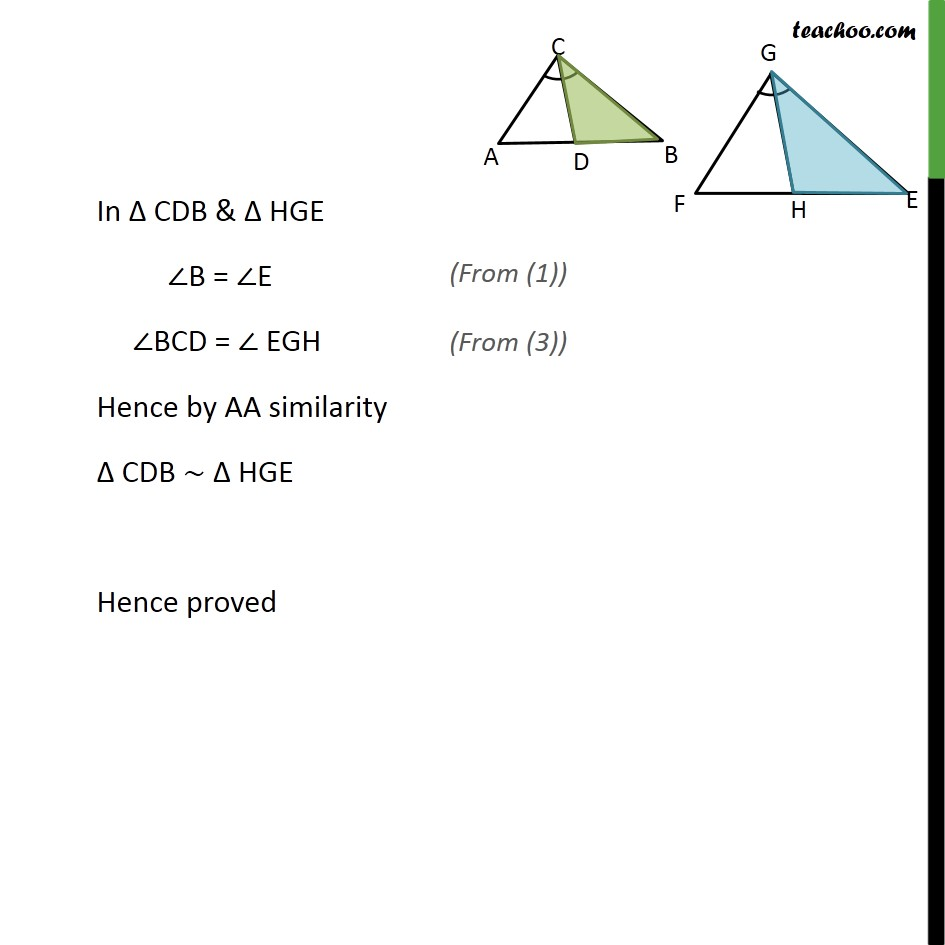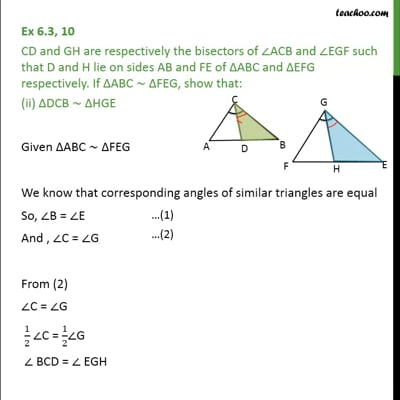This video is only available for Teachoo black users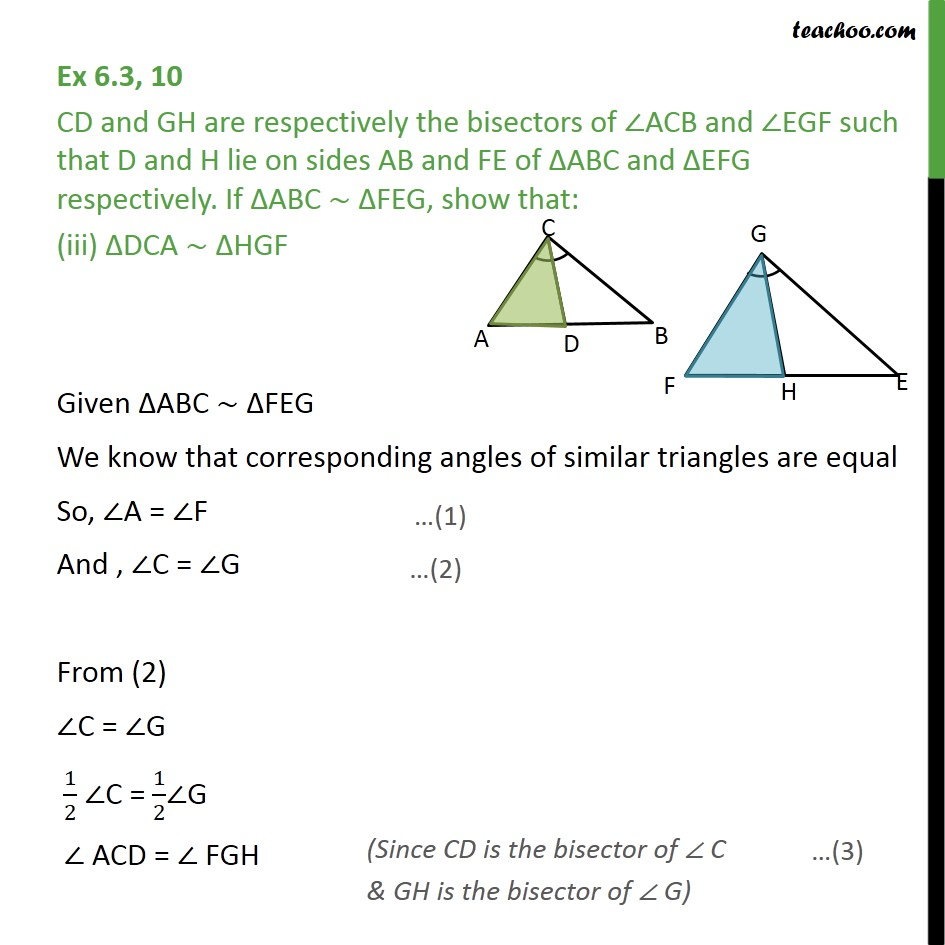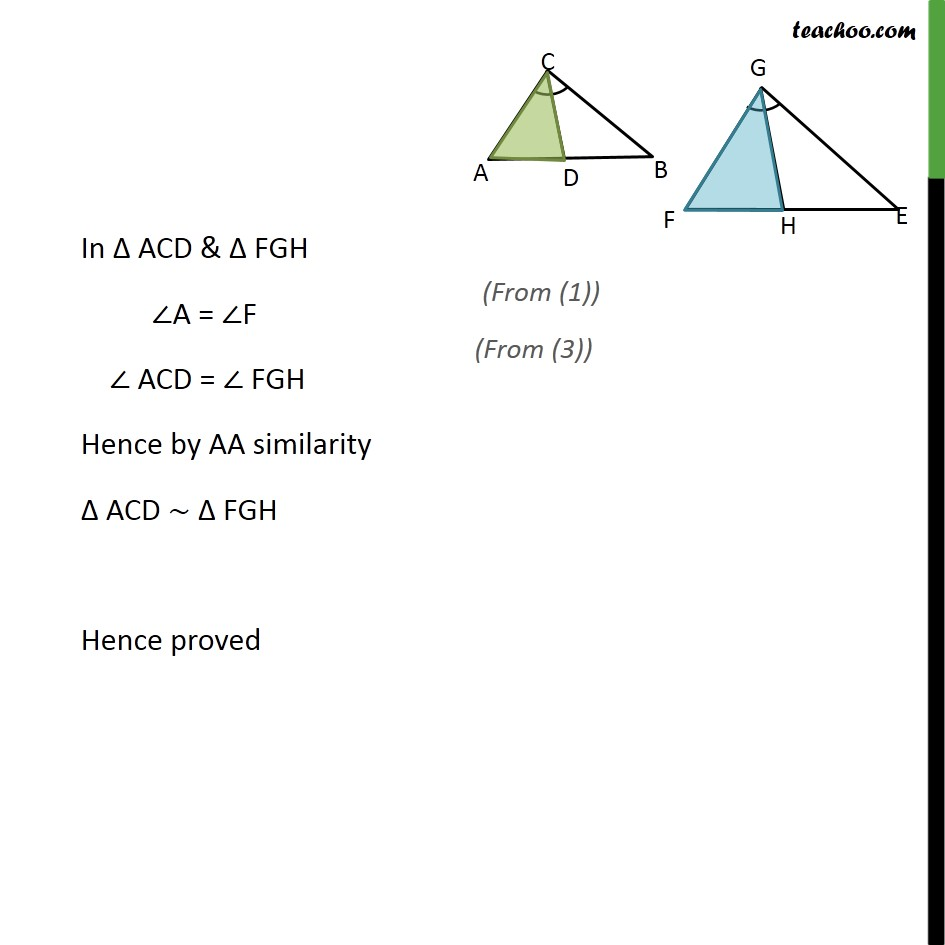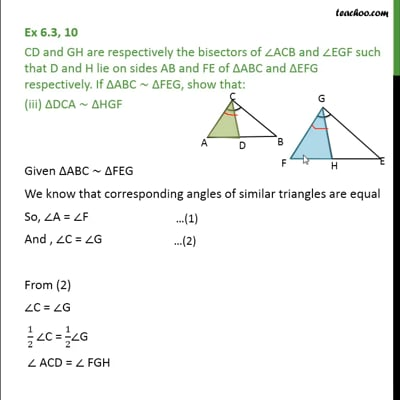This video is only available for Teachoo black users

### Transcript

Ex 6.3, 10 CD and GH are respectively the bisectors of ACB and EGF such that D and H lie on sides AB and FE of ABC and EFG respectively. If ABC FEG, show that: / = / Given: ACB and EGF respectively And , CD is the bisectors of ACB ACD = BCD = 1/2 ACB & GH is the bisectors of EGF FGH = EGH = 1/2 EGF & ABC FEG To Prove: / = / Proof: Given ABC FEG We know that corresponding angles of similar triangles are equal So, A = F And , C = G From (2) C = G 1/2 C = 1/2 G ACD = FGH In ACD & FGH A = F ACD = FGH Hence by AA similarity ACD FGH We know that corresponding sides of similar triangles are in proportion / = / Hence proved Ex 6.3, 10 CD and GH are respectively the bisectors of ACB and EGF such that D and H lie on sides AB and FE of ABC and EFG respectively. If ABC FEG, show that: (ii) DCB HGE Given ABC FEG We know that corresponding angles of similar triangles are equal So, B = E And , C = G From (2) C = G 1/2 C = 1/2 G BCD = EGH In CDB & HGE B = E BCD = EGH Hence by AA similarity CDB HGE Hence proved Ex 6.3, 10 CD and GH are respectively the bisectors of ACB and EGF such that D and H lie on sides AB and FE of ABC and EFG respectively. If ABC FEG, show that: (iii) DCA HGF Given ABC FEG We know that corresponding angles of similar triangles are equal So, A = F And , C = G From (2) C = G 1/2 C = 1/2 G ACD = FGH In ACD & FGH A = F ACD = FGH Hence by AA similarity ACD FGH Hence proved